# Difference between revisions of "Curie's law"

In a paramagnetic material Curie's law relates the magnetization of the material to the applied magnetic field and temperature.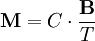$\mathbf{M} = C \cdot \frac{\mathbf{B}}{T}$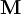$\mathbf{M}$ is the resulting magnetisation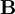$\mathbf{B}$ is the magnetic flux density of the applied field, measured in teslas$T$ is absolute temperature, measured in Kelvin.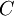$C$ is a material-specific Curie constant

This relation was discovered experimentally (by fitting the results to a correctly guessed model) by Pierre Curie.

## Simple Derivation (Statistical Mechanics)

A simple model of a paramagnet concentrates on the particles which compose it, call them paramagnetons. Assume that each paramagneton has a magnetic moment given by :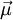$\vec{\mu}$. Energy of a magnetic moment in a magnetic field is given by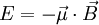$E=-\vec{\mu}\cdot\vec{B}$

To simplify the calculation, we are going to work with a 2-state paramagnet, that is, the particle can either align its magnetic moment with the magnetic field, or against it. No other orientations are possible,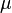$\mu$ and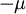$-\mu$. If so, then such particle has only two possible energies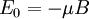$E_0 = - \mu B$

and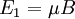$E_1 = \mu B$

With this information we can construct the partition function of one paramagneton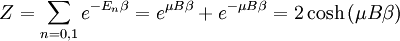$Z = \sum_{n=0,1} e^{-E_n\beta} = e^{ \mu B\beta} + e^{-\mu B\beta} = 2 \cosh\left(\mu B\beta\right)$

When one seeks the magnetization of a paramagnet, one is interested in the likelihood of a paramagneton to align itself with the field. In other words, one seeks the expectation value of orientation$\mu$.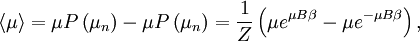$\left\langle\mu\right\rangle = \mu P\left(\mu_n\right) - \mu P\left(\mu_n\right) = {1 \over Z} \left( \mu e^{ \mu B\beta} - \mu e^{ - \mu B\beta} \right),$

where the probability of a configuration is given by its Boltzmann factor, and the partition function provides the necessary normalization for probabilities (so that the sum of all of them is unity.) A standard procedure is to express this as a derivative with respect to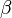$\beta$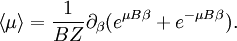$\left\langle\mu\right\rangle = {1 \over B Z} \partial_{\beta} ( e^{ \mu B\beta} + e^{ - \mu B\beta} ).$

But now what is differenciated is nothing but the partition function again. As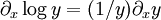$\partial_x \log y= (1/y) \partial_x y$, we can write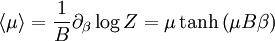$\left\langle\mu\right\rangle = {1 \over B } \partial_{\beta} \log Z = \mu \tanh\left(\mu B\beta\right)$

This is magnetization of one paramagneton, total magnetization of the solid is given by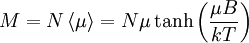$M = N\left\langle\mu\right\rangle = N \mu \tanh\left({\mu B\over k T}\right)$

The formula above is known as the Langevin Paramagnetic equation. Pierre Curie found an approximation to this law that applies to the reasonably high temperatures and low magnetic fields used in his experiments. Let's see what happens to the magnetization as we specialize it to large$T$ and small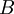$B$. As temperature increases and magnetic field decreases, the argument of hyperbolic tangent decreases. Another way to say this is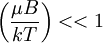$\left({\mu B\over k T}\right) << 1$

this is sometimes called the Curie regime. We also know that if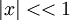$|x|<<1$, then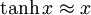$\tanh x \approx x$

so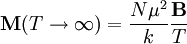$\mathbf{M}(T\rightarrow\infty)={N\mu^2\over k}{\mathbf{B}\over T}$

Q.E.D.

## More Involved Derivation (Statistical Mechanics)

A more involved treatment applies when the paramagnetons are supposed to rotate freely. In this case, their position will be determined by their angles in spherical coordinates, and the energy for one of them will be: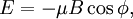$E = - \mu B\cos\phi,$

where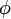$\phi$ is the angle between the magnetic moment and the magnetic field (which we take to be pointing in the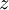$z$ coordinate.) The corresponding partition function is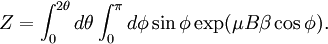$Z = \int_0^{2\theta} d\theta \int_0^{\pi}d\phi \sin\phi \exp( \mu B\beta \cos\phi).$

We seeing there is no dependence on the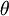$\theta$ angle, and we can change variables to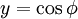$y=\cos\phi$ to obtain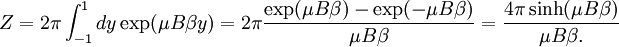$Z = 2\pi \int_{-1}^ 1 d y \exp( \mu B\beta y) = 2\pi{\exp( \mu B\beta )-\exp(-\mu B\beta ) \over \mu B\beta }= {4\pi\sinh( \mu B\beta ) \over \mu B\beta .}$

Now, the expected value of the$z$ component of the magnetization (the other two are seen to be null, as the should) will be given by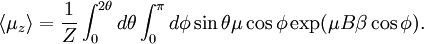$\left\langle\mu_z \right\rangle = {1 \over Z} \int_0^{2\theta} d\theta \int_0^{\pi}d\phi \sin\theta \mu\cos\phi \exp( \mu B\beta \cos\phi).$

Again, we see this can be written as a differenciation of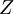$Z$: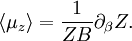$\left\langle\mu_z\right\rangle = {1 \over Z B} \partial_\beta Z.$

Carrying out the derivation we find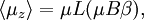$\left\langle\mu_z\right\rangle = \mu L(\mu B\beta),$

where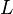$L$ is the Langevin function: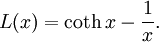$L(x)= \coth x -{1 \over x}.$

This function is not singular for small$x$, since the two singular terms cancel each other. In fact, its behavior for small arguments is the same as the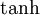$\tanh$ function, so the limit above also applies in this case.

## Applications

It is the basis of operation of magnetic thermometers, that are used to measure very low temperatures.

This entry contains material taken from this wikipedia entry.